• 基于已知输入 (u) 和输出 (y)，该函数返回脉冲响应的系数以及原始信号与重建信号的信噪比（以 dB 为单位）。 鼓励用户尝试各种响应长度并保留最短的响应长度，从而产生可接受的噪声信号比。 参考：Karel J. ...matlab
• 已知系统模型、初始状态、输入，三种方法求输出 用matlab进行计算微分方程解结果一样。 >> y=dsolve('Dy+y=1','y(0)=0','t') y = 1 - exp(-t) 值得考虑的是在输入u为序列，而不是t的显式函数，如何求...

已知系统模型、初始状态、输入，三种方法求输出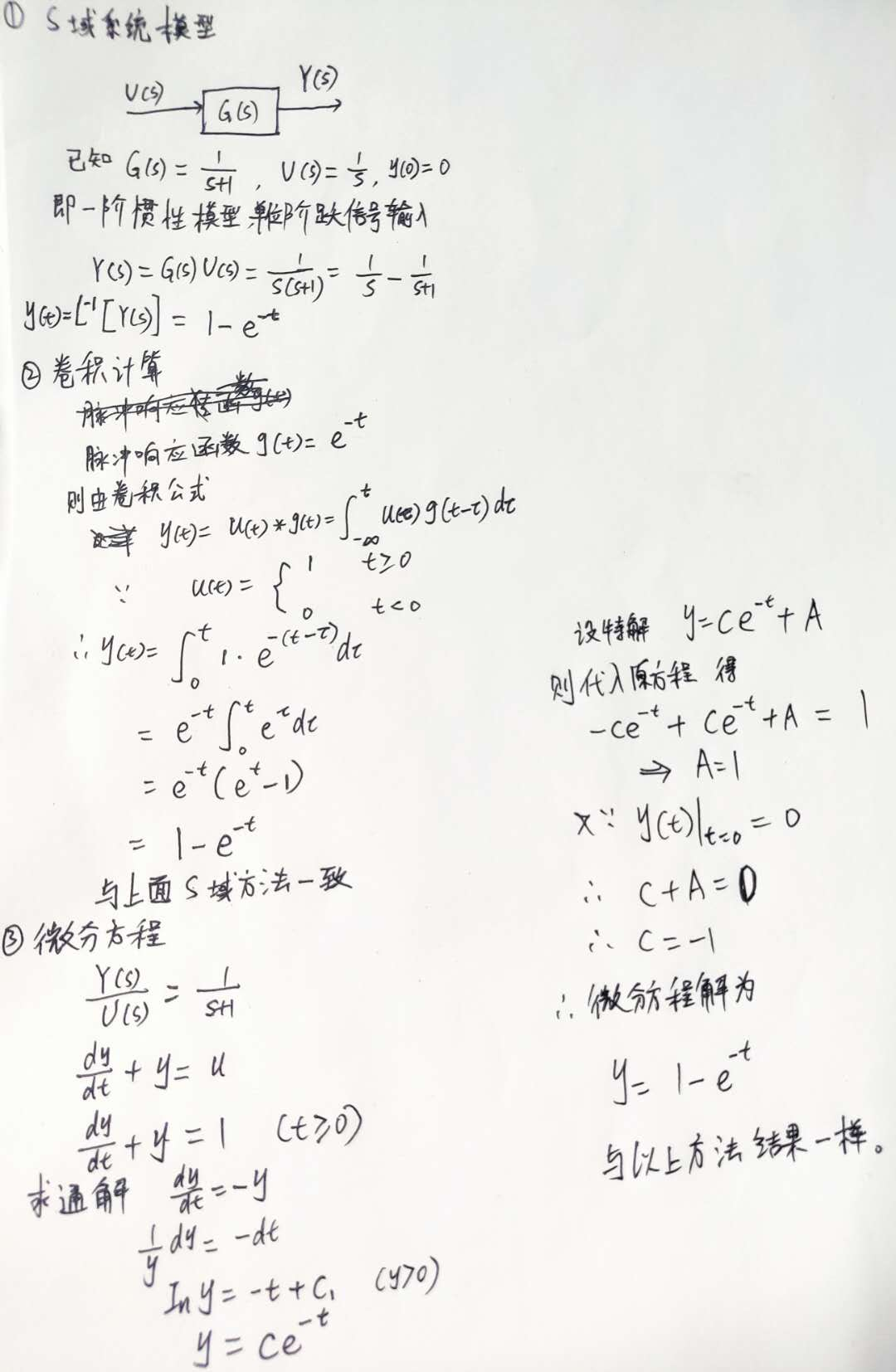用matlab进行计算微分方程解结果一样。

>> y=dsolve('Dy+y=1','y(0)=0','t')

y =

1 - exp(-t)

值得考虑的是在输入u为序列，而不是t的显式函数时，如何求输出序列？目前思考的是用卷积方法应该可以。
目前在matlab里面用微分方程尝试了对任意时间序列ut求输出序列，结果不对。matlab自带的lsim函数很好的解决了这个问题。想用在python里需要自己写代码，阅读lsim函数改写也并不容易。在尝试解决，期待早点解决。

展开全文算法 matlab 控制器
• C语言 输入和输出

千次阅读 2018-09-28 21:59:26
一.输入输出 单个字符的输入输出—getchar()...何为单个字符的输入输出？ 记住三条命令的使用条件： 1—char c ; 2—c = getchar(); 3—putchar©; 接招看题001：从键盘上输入一个大写的字母，并将...

开门见山，牛刀小试：
算法：

1. 首先，C=2PIR。S=PIRR。
2. 其次，定义float变量C，S，R，PI，共四个。
3. 然后，你要把R的数值告诉计算机，让计算机接受数据并处理。
4. 最后，计算机在屏幕上输出结果。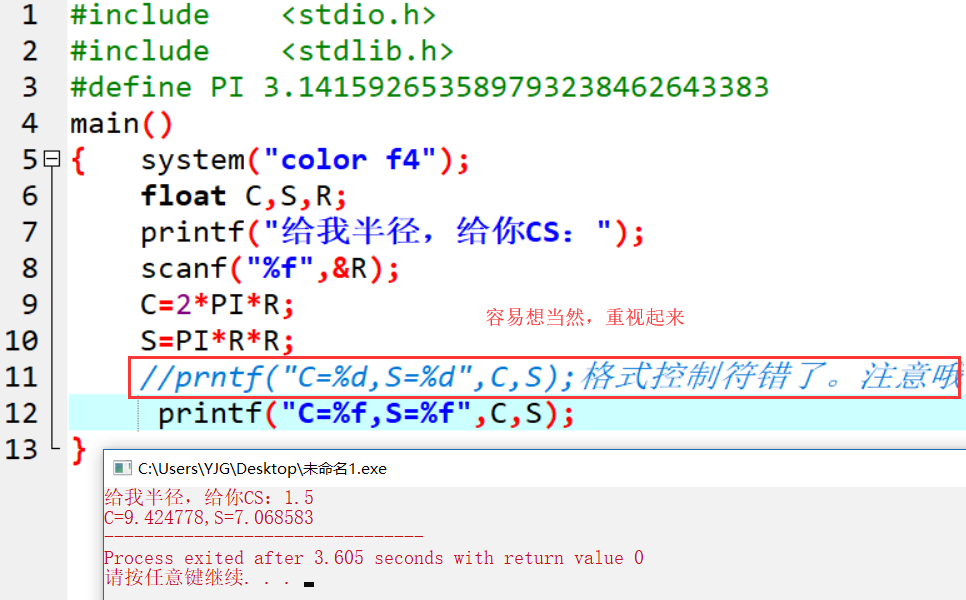#include	<stdio.h>
#include	<stdlib.h>
#define PI 3.141592653589793238462643383
main()
{	system("color f4");
float C,S,R;
printf("给我半径，给你CS：");
scanf("%f",&R);
C=2*PI*R;
S=PI*R*R;
//prntf("C=%d,S=%d",C,S);格式控制符错了。注意哦
printf("C=%f,S=%f",C,S);
}

实战案例02.类型转换字符c的使用。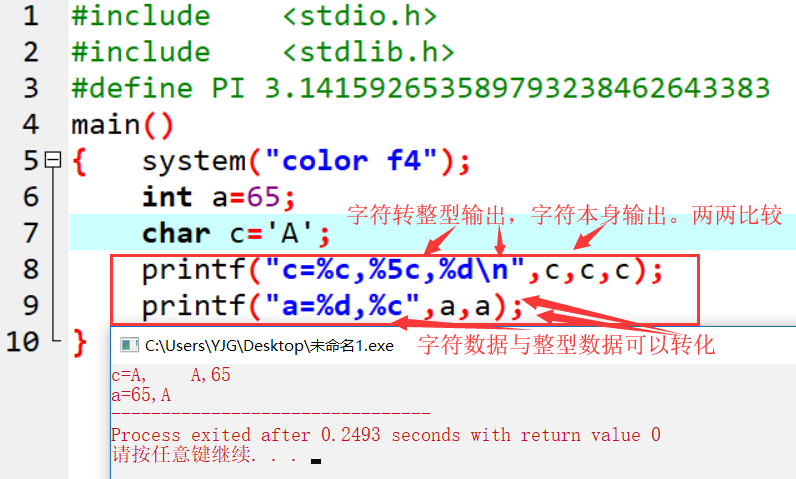#include	<stdio.h>
#include	<stdlib.h>
#define PI 3.141592653589793238462643383
main()
{	system("color f4");
int a=65;
char c='A';
printf("c=%c,%5c,%d\n",c,c,c);
printf("a=%d,%c",a,a);
}

需要强调的是：
在C语言中，整数可以用字符形式输出，字符数据也可以用整数形式输出。
将整数用字符形式输出时，系统首先求该数与256的余数，然后将余数作为ASCII码，转换成相应的字符输出。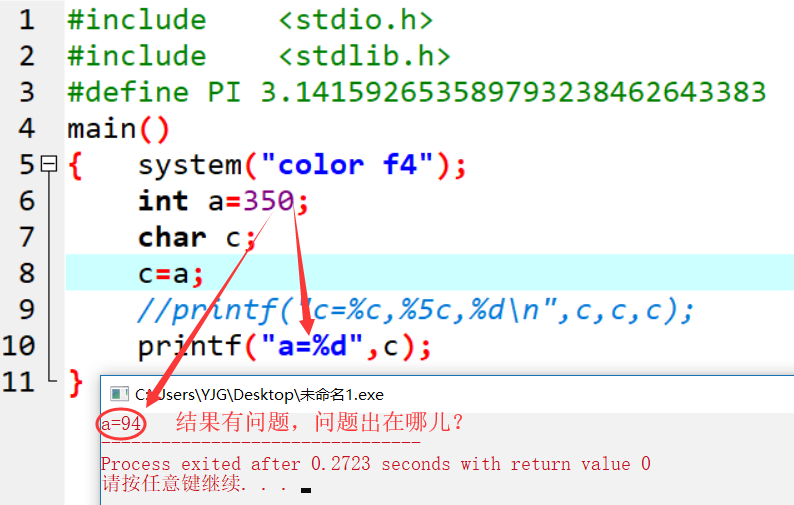** 数据的格式化的屏幕输出**
即printf(“格式控制转换符+需要的原样输出字符”,变量或表达式)
格式控制转换符即%d,%f,%c之类的格式控制符的统称。
变量或表达式之间要用逗号隔开。printf()函数用于显示格式化输出，它用变量的值来替换格式符，像这样：图解如下：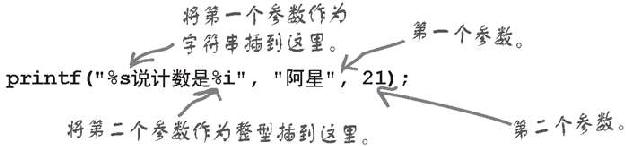一.输入和输出

1. 单个字符的输入和输出—getchar()和putchar()
相关知识：
何为字符常量？
即用单引号(’ ')括起来的字符。例如：'a’是字符常量，a是标识符。例如’5’也是字符常量，5是常数。
何为单个字符的输入输出？
记住三条命令的使用条件：

1---char c ;
2---c = getchar();
3---putchar();

接招看题001：从键盘上输入一个大写的字母，并将它转化成小写的，在屏幕上输出。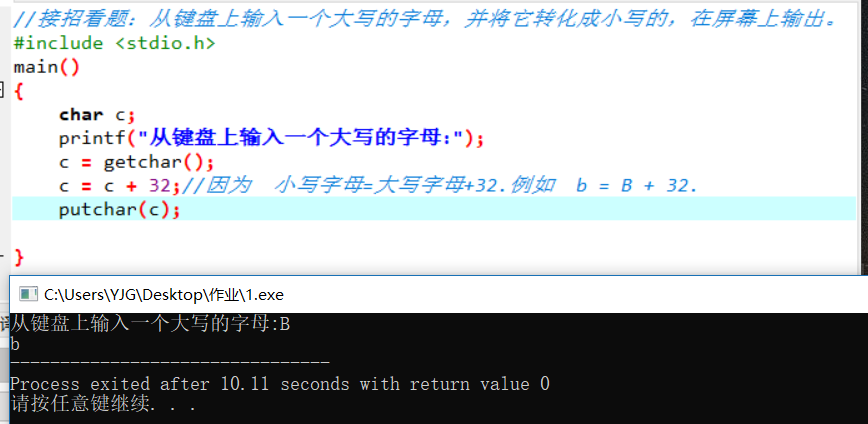知识补丁：何为字符？
通俗一点讲，字符就是键盘上所有的按键。深入一点讲，人们平时交流的语言，书籍上记载的文字，这些都叫字符。因为字符的本质是传递信息，字符是有意义的字母符号。在中国它主要是以汉字的形式存在，在英国主要是以英语的形式存在。一种符号，只要它能够向人们表达特定的信息，都可以称为字符。
何为特殊的字符常量？
即转义字符。例如’\n’表换行；’\t’表制表位；’\r’表回车。等等。

2. 数据的格式化的屏幕输出
即printf(“格式控制转换符+需要的原样输出字符”,变量或表达式)
格式控制转换符即%d,%f,%c之类的格式控制符的统称。
变量或表达式之间要用逗号隔开。printf()函数用于显示格式化输出，它用变量的值来替换格式符，像这样：图解如下：当调用printf()时，可以包含任意数量的参数，但确保每个参数都要有一个对应的%格式符。一一对应的。

接招看题002：从键盘输入一个大写的字母，将其转换为小写的英文字母后，在屏幕上输出转换后的小写字母及其对应的十进制的ASCII码值。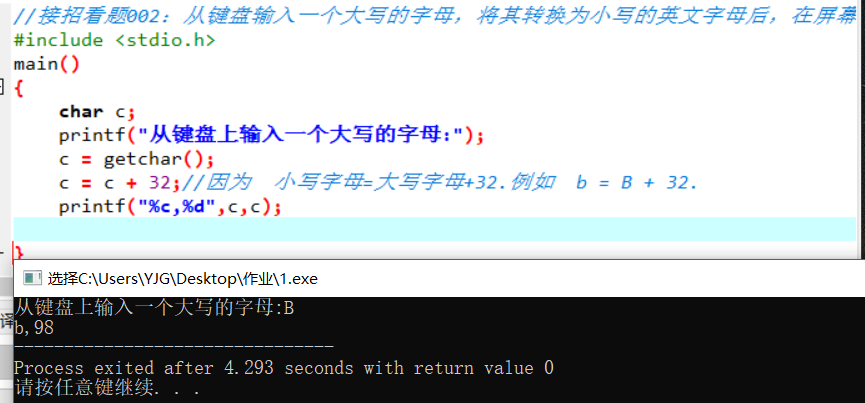知识补丁：
为什么要用到printf函数来输出而不是用putchar()来输出呢？
即二者的使用条件是什么？
putchar()是字符型输出，只输出字符型数据；
printf()函数可以输出任意类型的数据，既可以输出字符型数据，也可以输出整型数据，还可以输出浮点型数据，等等。
我们可以根据两者输出数据类型的差异，加上题目，项目的要求，合理使用输出方式。
3. 数据的格式化的键盘输入
即scanf(“格式控制转换符+分隔符”,参数地址表)
格式控制转换符即%d,%f,%c之类的格式控制符的统称。
参数地址表，例如&a,&b,%c，即参数地址表=取地址运算符&+变量a,b,c。由取地址运算符&+变量组成。
接招看题003：scanf()函数输入数据演示。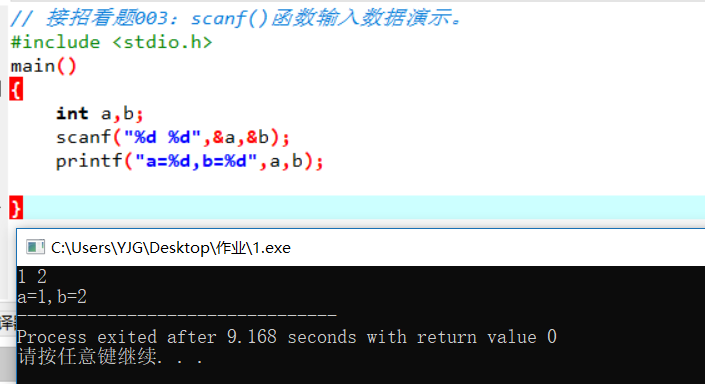展开全文• 数字信号处理matlab训练【3】1.LSI系统的转移函数1.1 频率响应1.2 转移函数1.3 差分方程1.4 卷积关系1.5 系统的转移函数$H(Z)$...对于一个线性不变离散时间系统（如下图所示），我们在之前就已经接触到了4种不同的...

1.LSI系统的转移函数

对于一个线性时不变离散时间系统（如下图所示），我们在之前就已经接触到了4种不同的描述方法。1.1 频率响应

H ( e j ω ) = ∑ n = 0 ∞ h ( n ) e − j ω n H(e^{j\omega})=\sum_{n=0}^{\infty}h(n)e^{-j\omega n}

1.2 转移函数

H ( z ) = ∑ n = 0 ∞ h ( n ) z − n H(z)=\sum_{n=0}^{\infty}h(n)z^{-n}

1.3 差分方程

y ( n ) = − ∑ k = 1 N a ( k ) y ( n − k ) + ∑ r = 0 M b ( r ) x ( n − r ) y(n)=-\sum_{k=1}^Na(k)y(n-k)+\sum_{r=0}^Mb(r)x(n-r)

1.4 卷积关系

y ( n ) = x ( n ) ∗ h ( n ) = ∑ k = − ∞ ∞ x ( k ) h ( n − k ) y(n)=x(n)*h(n)=\sum_{k=-\infty}^\infty x(k)h(n-k)
上边的4种方法从不同的角度描述了一个LSI系统的物理特性，他们之间有着密切的联系，其联系的纽带就是系统的单位冲激响应 h ( n ) h(n)

1.5 系统的转移函数 H ( Z ) H(Z)

对上边的第三式两边取Z变换，得
Y ( z ) = − Y ( z ) ∑ k = 1 N a ( k ) z − k + X ( z ) ∑ r = 0 M b ( r ) z − r Y ( z ) [ 1 + ∑ k = 1 N a ( k ) z − k ] = X ( z ) [ b ( 0 ) + ∑ r = 1 M b ( r ) z − r ] Y(z)=-Y(z)\sum_{k=1}^Na(k)z^{-k}+X(z)\sum_{r=0}^Mb(r)z^{-r}\\ \quad \\Y(z)[1+\sum_{k=1}^Na(k)z^{-k}]=X(z)[b(0)+\sum_{r=1}^Mb(r)z^{-r}]
对上边的式子进行整理可得
H ( z ) = Y ( z ) X ( z ) = ∑ r = 0 M b ( r ) z − r 1 + ∑ k = 1 N a ( k ) z − k H(z)=\frac{Y(z)}{X(z)}=\frac{\sum_{r=0}^Mb(r)z^{-r}}{1+\sum_{k=1}^Na(k)z^{-k}}
上式 H ( z ) H(z) 就被称为系统的转移函数。它即可以定义为系统单位抽样响应 h ( n ) h(n) 的z变换，也可以 定义为系统的输出、输入Z变换之比。

2.matlab编程训练，求系统的阶跃响应

我们之前学过的，如果已经知道系统的 h ( n ) h(n) ,由于 y ( n ) = x ( n ) ∗ h ( n ) y(n)=x(n)*h(n) ,则我们可以轻松的使用conv函数将其求得，但是如果我们不知道 h ( n ) h(n) ,已知 Y ( z ) Y(z) X ( z ) X(z) ,需要求 y ( n ) y(n) ，则我们可以根据公式，
y ( n ) = − ∑ k = 1 N a ( k ) y ( n − k ) + ∑ r = 0 M b ( r ) x ( n − r ) = b ( 1 ) x ( n ) + b ( 2 ) x ( n − 1 ) + . . . + b ( n b + 1 ) x ( n − n b ) − a ( 2 ) y ( n − 1 ) − . . . − a ( n a + 1 ) y ( n − n a ) y(n)=-\sum_{k=1}^Na(k)y(n-k)+\sum_{r=0}^Mb(r)x(n-r)\\ \quad \\=b(1)x(n)+b(2)x(n-1)+...+b(n_b+1)x(n-n_b)\\ \quad \\-a(2)y(n-1)-...-a(n_a+1)y(n-n_a)
求取我们的 y ( n ) y(n) ,使用matlab内的一个函数 y = f i l t e r ( b , a , x ) y=filter(b,a,x) 其中 x 、 y 、 a 和 b x、y、a和b 都是向量。

2.1 编程实训

已知一个系统的转移函数如下所示：
H ( z ) = 0.001836 + 0.007344 z − 1 + 0.011016 z − 2 + 0.007374 z − 3 + 0.001836 z − 4 1 − 3.0544 z − 1 + 3.8291 z − 2 − 2.2925 z − 3 + 0.55075 z − 4 H(z)=\frac{0.001836+0.007344z^{-1}+0.011016z^{-2}+0.007374z^{-3}+0.001836z^{-4}}{1-3.0544z^{-1}+3.8291z^{-2}-2.2925z^{-3}+0.55075z^{-4}}
求该系统的阶跃响应。

%%转移函数
clc;
clear;
x=ones(100,1);
%定义阶跃输入
t=1:100;
b=[0.001836,0.007344,0.011016,0.007374,0.001836];
%定义向量b
a=[1,-3.0544,3.8291,-2.2925,0.55075];
%定义向量a
y=filter(b,a,x);
%得到y(n)
hold on
h1=plot(t,x,'b');
h2=plot(t,y,'r');
hold off
%画图结束
legend([h1,h2],'阶跃输入','阶跃响应');
%添加标注

其运行结果为：2.2 求取系统的单位抽样响应h(n)

在我们得到上边的情况下，如果我们想要得到该系统的单位抽样响应，编辑mtalab代码如下：

[h,t]=impz(b,a,40);
%h(n)的长度为N=40
stem(t,h,'.');
%绘制离散的h(n)
grid on;

其显示结果如下所示：2.3 求上述系统的频率响应

%%得到系统的频率响应
[H,w]=freqz(b,a,256,'whole',1);
%whole 指定计算的频率范围是0-Fs,此处我们设置Fs=1
Hr=abs(H);
%得到绝对值，也就是幅度值
plot(w,Hr,'r','linewidth',2);
%绘制图形，并且设置颜色和线宽
grid on;
%显示方格
xlabel('\omega /2\pi');
ylabel('|H(e^{j\omega})|');
title('幅频响应')
%坐标轴设置

输出图像如下所示：3.参考文章

数字信号处理理论、算法与实现（第三版）

展开全文matlab LSI系统
• 结论1 :给定单输入,单输出线性不变系统输入输出描述 m=n，即系统为真情形 m&lt;n，即系统为严真情形 例1：给定的系统的输入-输出描述为：m&lt;n   则系统的状态空间描述为  ...

由输入输出描述导出状态空间描述

对于单输入，单输出线性时不变系统,其微分方程描述

其传递函数描述

可以导出其状态空间描述为

结论1 :给定单输入,单输出线性时不变系统的输入输出描述

• m=n，即系统为真情形

• m<n，即系统为严真情形

• 例1：给定的系统的输入-输出描述为：m<n

则系统的状态空间描述为

• 例2：给定的系统的输入-输出描述为：m=n

则系统的状态空间描述为

• m=0情形

• m≠0情形

结论3 :给定单输入单输出线性时不变系统的传递函数描述为：

其极点即分母方程的根 为两两互异实数，则对应的状态空间描述可按如下两类情形导出：

• m<n，即系统为严真情形

对应的状态空间描述为

• m=n，即系统为真情形

​​​​​​​

对应的状态空间描述为

展开全文• 本文研究了在发送端已知信道均值或协方差信息情况下单用户多输入输出(MIMO)系统的功率分配问题.发送端已知信道均值信息,信道建模为非相关莱斯信道;发送端已知协方差信息,信道建模为相关瑞利信道.通过最大化...
• Matlab之Kalman：用线性系统状态方程，通过系统输入输出观测数据，对系统状态进行最优估计的算法 目录 问题探究 卡尔曼滤波及数据滤波 代码实现 问题探究 用线性系统状态方程，通过系统输入输出观测...
• 状态空间描述控制理论传递函数之间存在什么关系呢？ 状态空间描述为一种内部描述，为了状态空间对应的输出表达，在此探讨如何从状态空间表达式导出相应的传递函数表达式。 对于最一般形式的状态空间表达式： ...
• 【简答题】编写程序 sum.py ,输入任意大的自然数,输出各位数字之。【简答题】编写程序 ex3.py 实现功能:有一段英文文本,其中有单词连续重复了 2 次,编写程序检查重复的单词并只保留一个。例如文本内容为“ This is...
• 1系统标准输入文件是指(.doc

千次阅读 2021-05-20 13:21:44
1系统标准输入文件是指(PAGE 172...硬盘【答案】A【说明】键盘系统的标准输入文件，显示器是系统的标准输出文件。2．文件类型是一个( )。A．数组B．指针C．结构体D．地址【答案】C【说明】文件类型是系统定义的结构...
• c语言格式输入与输出

千次阅读 2021-05-20 14:30:06
putchar()函数getchar()函数只能输出输入一个字符，若要同时输入多种数据类型的数据，则可以使用格式输入输出函数。这种函数不但能输入输出各种类型的数据，而且还可以控制数据输入输出时每个数据的输入输出格式...
• 习 题5-1 已知单位反馈系统的开环传递函数，试绘制其开环极坐标图开环对数频率特性。(1)(2)(3)(4)5-2 设单位反馈系统的开环传递...5-4 已知图示RLC网络，ω=10rad/s系统的幅值A=1相角=-90°，试求其传递函数...
• 第五章 输入/输出 习题 知识点小记 I/O设备可分为：块设备字符设备 块设备：把信息存储在固定大小的块种妹妹个快有自己的地址。基本特征是每个块都能独立于其他块而读写。如硬盘、蓝光光盘USB盘。 ...
• 开环控制系统和闭环控制系统在日常生活中的例子1开环控制...指令输入到计算机控制系统之中,带动步已知单位负反馈控制系统的开环传递函数为G(s)=10/s+10,求r(t)=1输出y(t)求详细解法Y(s)=R(s)*G(s)/(1+G(s))=(1...
• 一、用微分差分方程描述的因果线性不变系统1、一类极为重要的连续时间系统是其输入输出关系用线性常系数微分方程描述的系统。这种形式的方程可以用来描述范围官广泛的系统和屋里现象。2、一类极为重要的离散时间...信号与系统
• Python-入门中的入门——打印日历题干大意代码语法易错点整理输入输出格式for循环的range 历程： 大一上半年C++书中的案例，连注释带上代码一共二百行，当时自己C++写了足足200行才解决。后来19年元旦自学python拿起...Python 输入输出 for循环用法 打印日历
• 机器学习三个部分：输入、算法、输出输入：驱动机器学习的数据 输入是训练算法需要的数据集。从源代码到统计数据，数据集可以包含任何东西： GSA / data（美国总务管理局数据）：...apache spark
• 已知单位负反馈控制系统的开环传递函数为G=K/S,试根据劳思确定系统稳定的τ^令1+G(s)=0,得到特征方程D(s)=S(τS+1)(2S+1)+k(s+1)=2τS^3+(2+τ)s^2+(k+1)s+k.routh判据：s^3 2τ k+1s查看详细已知单位负反馈系统的...
• Hello，欢迎做客我的博客。 这里会介绍关于机器人的方方面面，希望对你有所帮助。...MATLAB的System Identification Toolbox（系统辨识工具箱），其中的Nonlinear Grey-Box Models（非线性灰箱建...
• 输入和输出解释： 工具解释： 1 1 制定项目章程 （项目整合） 制定一份正式批准项目或阶段的文件，并记录能反映干系人需要期望的初步要求的过程 1、项目工作说明书 2、商业论证 3、...项目管理
• 第五章 输入/输出 1.芯片技术的进展已经使得将整个控制器包括所有总线访问逻辑放在一个便宜的芯片上成为可能。这对于图1-5的模型具有什么影响？ 答：（题目有问题，应该是图1-6）在此图中，一个控制器有两个...操作系统
• 地址总线中的 条线可用于I/O寻址,形成 的输入输出地址空间,地址范围为 ;PC机中用了 条地址线进行I/O操作,其地址空间为 ,可寻址范围为 。 2．实现主机与外设之间同步需要解决的基本问题有二，一为测试 编程 存储 c
• 中国大学MOOC: 编程计算下面的分段函数，根据从键盘输入的x值，在屏幕上输出y值。答：else if (x == 0)具有明显方向性足够强度，用来确定画面主调和气氛的是：答：主光法律道德是维护社会秩序的两种基本手段，...
• } catch (IOException e) { System.out.println("系统错误1!"); e.printStackTrace(); } catch (Exception e) { System.out.println("所输入不为数字!"); e.printStackTrace(); } finally { try { is.close(); br....
• 百度百科对概念进行了解释：稳态响应是指足够长的时间之后，系统对于固定的输入，有了一个较为稳定的输出。在某一输入信号的作用后，时间趋于无穷大时系统输出状态称为稳态。下面就一起来体会...
• 数字信号处理matlab——系统响应和系统稳定

千次阅读 多人点赞 2021-07-02 09:30:06
检查系统稳定性最普遍的做法是：输入单位阶跃序列，n→∞，系统输出趋近于一个常数，那么系统是稳定的 例一 给定一个差分方程 y(n)=0.05x(n)+0.05x(n-1)+0.9y(n-1) 输入信号x(n)=R8(n) 求x(n)的系统响应，画出波形...信号处理 数字信号处理 卷积 idft
• 线性时不变系统

千次阅读 2020-08-06 23:15:23
一、离散时间线性不变系统：卷积 一）、用脉冲表示离散时间信号 1、如何把任何离散时间时间信号看成由离散时间单位脉冲构成的关键是：要把一个离散时间信号当成一串单个脉冲来想象。 2、离散时间单位脉冲序列的...信号处理
• 系统的分类 ...系统跳过了电路的具体结构，只考虑输入(激励e(t)e(t)e(t))和输出（响应r(t)r(t)r(t)）的关系。 其中系统函数H[n]H[n ]H[n]是表征系统固有特性的函数，因此与外加激励毫无关系，只...
• 接下来先研究一下打开的文件描述符,此前一直采取的是让读进程读取一些数据然后直接退出的方式,并假设linux会把清理文件当作是在进程结束应该做的工作的一部分. 但大多数从标准输入读取数据的程序采用的确实与此前...
• 从大到小： #include using namespace std; int main() {  int a; int n; cin>>n; for(int i=0;i { cin>>a[i]; } for(int i=0;i { for(int j=0;j { if(a[j] swap(a[j],a[j+1]);...for(int i...